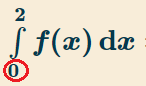# Integral Bounds / Limits of Integration

## What are Integral Bounds?

Integral bounds , also called limits of integration, define the area that you’ll be integrating.

## Upper Bounds and Lower Bounds

An integral has two bounds: a lower bound and an upper bound. If you’re given an integral, you’ll be integrating between these two bounds.

The lower bound is where you start integrating. You’ll find it underneath the integral symbol:The upper bound is the line at which you stop integrating. It’s above the integral symbol:For the above example, you would say that the bounds of integration are 0 and 2.

## How to Find Limits of Integration

If you’re given a series of functions and asked to find the bounded area (that the functions contain), the easiest way to find the limits of integration is to graph the functions.

For example, let’s say you were asked to find the bounded area of:

• y = 5 – x
• y = x
• y = 4 – 3x

On a graph, the functions look like this:Limits of integration (black dots) are easy to see if you graph the functions.
You can use your graphing calculator (I used the online graphing calculator at HRW) to zoom in to where the lines meet. Note that in this example, the area is bounded by three functions, so you’ll need to perform more than one integration to find the solution. You can see a simple example of this concept here: Area between two curves.

## Definite Integrals and Bounds

Integral bounds only apply to definite integrals.

If you have a continuous function (i.e. one that extends towards infinity), you must put bounds on the function in order to integrate it. When you place these lower and upper bounds, it becomes a definite integral. An integral without bounds is called an indefinite integral.

## How to find the upper limit of an integral

When you solve for the upper limit of an integral, you’re solving for a definite integral with an upper bound.Integral showing a lower limit of -1 and an upper limit of 1.

## Steps

Step 1: Identify the function in question. In an integral, this is the value in between the integral symbol and the integration constant (usually denoted as ‘dx’ or perhaps ‘dy’). As an example, we’ll name the function to be something simple such as ‘f(x) = 4x’.
Step 2: Identify the calculus limits of the integral. These values are typically denoted at the top and bottom of the integral sign. The upper bound is the value up top and the lower bound is the value at the bottom of the symbol. We’ll allow the upper bound to be 2 while the lower bound is 1.
Step 3: Perform the integration of the function using indefinite integral rules. For f(x) = 4x, raise the power of the variable by one and divide the entire function by the new exponent of the variable. For example, the integral of f(x) = 4x becomes 2x2.
Step 4: Insert the upper bound of the integral into the newly integrated function. We replace our variable with the previously identified upper bound (which we valued at 2). Our integrated function then becomes f(x) = 2(2)2, where the value in the parentheses is the upper bound that replaced the variable.
Step 5: Perform the mathematical computation. Do not forget the order of operations—your answer could result in something very different if you fail to follow this step!
f(x) = 2(2)2 = 2(4) = 8. So for the function f(x) = 4x, integrating it with an upper bound of 2 will result in the value 8.

That’s it!

## Issues

Even if an integral has bounds, that doesn’t always mean there’s an analytical solution to the integral. For example, there may be an essential discontinuity between the lower bound and the upper bound. It’s always a good idea to graph a function before you try and integrate it; in most cases, you can avoid this problem. That said, well-behaved, continuous functions are usually integrable (King & Mody, 2010).

## References

King, M. & Mody, N. (2010). Numerical and Statistical Methods for Bioengineering: Applications in MATLAB. Cambridge University Press.

CITE THIS AS:
Stephanie Glen. "Integral Bounds / Limits of Integration" From StatisticsHowTo.com: Elementary Statistics for the rest of us! https://www.statisticshowto.com/integrals/integral-bounds/